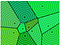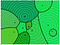# Discriminant Analysis- Linear and Gaussian

Now Logistic Regression and Multinomial Regression are called Discriminant learning algorithms which learn p(y|x) directly.

Naive bayes and linear/quadratic discriminant analysis are called Generative learning algorithms that try to model p(x|y) and p(y). They use Bayes rule to derive p(y|x).

Discriminant Analysis

Discriminant analysis seeks to model the distribution of X in each of the classes separately. Bayes theorem is used to flip the conditional probabilities to obtain P(Y|X). The approach can use a variety of distributions for each class. The techniques discussed will focus on normal distributions

Linear Discriminant Analysis:

With linear discriminant analysis, there is an assumption that the covariance matrices Σ are the same for all response groups.

For p(no. of independent variables)= 1:

Recall the pdf for the Gaussian distribution:

Then

where πk=P(Y=k).

Simplify by taking logs and simplifying

Since the objective is to maximize, remove all constants (terms that do not depend on kk) to obtain the discriminant score

Assign xx to the class with the largest discriminant score.

For p > 1:

The pdf for the multivariate Gaussian distribution:

The discriminant function is

This method assumes that the covariance matrix Σ is the same for each class.

Estimate the model parameters using the training data.

Compute the class posterior probabilities with the discriminant function

Decision boundaries are pictorially represented like[When you have many classes, their LDA decision boundaries form a classical Voronoi diagram if the priors ⇡C are equal. All the Gaussians have the same width.]

Note: That the decision function

in case of two cases, when subtracted for two classes give

gives decision boundary when w.x +a = 0 .[The effect of “w · x + a” is to scale and translate the logistic fn in x-space. It’s a linear transformation.]

The translation of posterior probabilities to logistic/ sigmoid function is

In quadratic discriminant analysis, do not make the assumption that the covariance matrix Σk is the same for each class.

This changes the discriminant function to

The decision boundaries are pictorially represented asf [The feature space gets partitioned into regions. In two or more dimensions, you typically wind up with multiple decision boundaries that adjoin each other at joints. It looks like a sort of Voronoi diagram. In fact, it’s a special kind of Voronoi diagram called a multiplicatively, additively weighted Voronoi diagram.]

Please Notes we might get coefficients are an output while implementing LDA, which simply means that coeff*variable value for each observation gives the decision rule, if there are only two class labels then,?

Please check NAIVE BAYES for generative algorithm for classification

# Logistic Regression vs. Discriminant Analysis vs. Naive Bayes

Best to use Logistic Regression:

• More robust to deviations from modeling assumptions (non-Gaussian features)

Best to use Discriminant Analysis:

• When the assumption that the features are Gaussian can be made
• More efficient than logistic regression when the assumptions are correct
• Works better than logistic regression when data is well-separated
• Popular for multinomial responses since it provides a low-dimensional view of data

Best to use Naive Bayes:

• Can make the assumption that features are independent (conditional on response)
• Despite strong assumptions, works well on many problems

Resources:

https://people.eecs.berkeley.edu/~jrs/189/lec/07.pdf

http://jennguyen1.github.io/nhuyhoa/statistics/Discriminant-Analysis-Naive-Bayes.html

Problem Solver, Data Science, Actuarial Science, Knowledge Sharer, Hardcore Googler

## More from Shaily jain

Problem Solver, Data Science, Actuarial Science, Knowledge Sharer, Hardcore Googler